# Oops...

This website is made for modern browsers. You are seeing this, because your browser is missing a feature or two. Please install the latest update or switch to a modern browser. See you soon.

# Gridshell joints

In this tutorial, we will model a joint in reference to one of a gridshell. Considerations of proper engineering are left out and the focus is solely on creating solids that are generated on aligned construction planes.

Operations on solids, like trimming or merging, are computationally intensive and will lock Grasshopper when parameters are changed upstream. Thus, the number of objects operated on should be limited. In this tutorial, this is done by creating a box that can be moved around and only the nodes inside the box are calculated.

## Grasshopper

The methods described in this tutorial expect that a surface for the gridshell is present and that there are segmented surfaces for each grid cell. To keep it simple, we will use a torus hereafter.

#### 0

##### Generate a gridshell surface and subdivide it

To generate a torus as base geometry, we create a Circle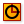Circle (Cir)
Curve  >  Primitive  >  Circle
Create a circle defined by base plane and radius.
Inputs
Plane (P)Base plane of circle
Outputs
Circle (C)Resulting circle
with a radius of 30 and then use End PointsEnd Points (End)
Curve  >  Analysis  >  End Points
Extract the end points of a curve.
Inputs
Curve (C)Curve to evaluate
Outputs
Start (S)Curve start point
End (E)Curve end point
to get a point on the circle. Next, we draw another circle on the XZ Plane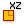XZ Plane (XZ)
Vector  >  Plane  >  XZ Plane
World XZ plane.
Inputs
Origin (O)Origin of plane
Outputs
Plane (P)World XZ plane
with a smaller radius of 15 and then use it as a section curve for Sweep1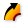Sweep1 (Swp1)
Surface  >  Freeform  >  Sweep1
Create a sweep surface with one rail curve.
Inputs
Rail (R)Rail curve
Sections (S)Section curves
Miter (M)Kink miter type (0=None, 1=Trim, 2=Rotate)
Outputs
Brep (S)Resulting Brep
and the first circle as rail curve.

To subdivide the surface of the torus, we first have to calculate the size of the grid cells. We do this with Dimensions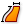Dimensions (Dim)
Surface  >  Analysis  >  Dimensions
Get the approximate dimensions of a surface
Inputs
Surface (S)Surface to measure
Outputs
U dimension (U)Approximate dimension in U direction
V dimension (V)Approximate dimension in V direction
and then use Division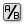Division (A/B)
Maths  >  Operators  >  Division
Mathematical division
Inputs
A (A)Item to divide (dividend)
B (B)Item to divide with (divisor)
Outputs
Result (R)The result of the Division
through 5 to get corresponding values. The component Divide Domain²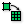Divide Domain² (Divide)
Maths  >  Domain  >  Divide Domain²
Divides a two-dimensional domain into equal segments.
Inputs
Domain (I)Base domain
U Count (U)Number of segments in {u} direction
V Count (V)Number of segments in {v} direction
Outputs
Segments (S)Individual segments
lets us divide a two-dimensional domain (we can directly plug in the torus) into segments according to our calculated values. We use Isotrim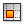Isotrim (SubSrf)
Surface  >  Util  >  Isotrim
Extract an isoparametric subset of a surface.
Inputs
Surface (S)Base surface
Domain (D)Domain of subset
Outputs
Surface (S)Subset of base surface
to subdivide the torus into segmented surfaces.

#### 1

##### Create a box to capture nodes with

To place a box on the surface of the torus, we have to find the coordinates for the box' base first. This is done with the component Evaluate Surface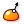Evaluate Surface (EvalSrf)
Surface  >  Analysis  >  Evaluate Surface
Evaluate local surface properties at a {uv} coordinate.
Inputs
Surface (S)Base surface
Point (uv){uv} coordinate to evaluate
Outputs
Point (P)Point at {uv}
Normal (N)Normal at {uv}
U direction (U)U direction at {uv}
V direction (V)V direction at {uv}
Frame (F)Frame at {uv}
on which input S is reparameterized. We can now use a MD Slider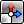MD Slider (MD Slider)
Params  >  Input  >  MD Slider
A multidimensional slider
to generate the coordinates and find a point on the surface. The box is created with Center Box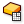Center Box (Box)
Surface  >  Primitive  >  Center Box
Create a box centered on a plane.
Inputs
Base (B)Base plane
X (X)Size of box in {x} direction.
Y (Y)Size of box in {y} direction.
Z (Z)Size of box in {z} direction.
Outputs
Box (B)Resulting box
and we use three Number Slider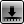Number Slider
Params  >  Input  >  Number Slider
Numeric slider for single values
to change the size of the box. Now, we can use all four sliders and the Rhino viewport to capture the desired number of nodes within this box. This selection will be used for further modeling of the solids.

#### 2

##### Isolate the captured nodes

To continue, we will further dismantle the grid cell surfaces with Deconstruct Brep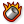Deconstruct Brep (DeBrep)
Surface  >  Analysis  >  Deconstruct Brep
Deconstruct a brep into its constituent parts.
Inputs
Brep (B)Base Brep
Outputs
Faces (F)Faces of Brep
Edges (E)Edges of Brep
Vertices (V)Vertices of Brep
and isolate the nodes that are within the box. For the latter, we use Point In Brep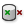Point In Brep (BrepInc)
Surface  >  Analysis  >  Point In Brep
Test whether a point is inside a closed brep
Inputs
Brep (B)Brep for inclusion test
Point (P)Point for inclusion test
Strict (S)If true, then the inclusion is strict
Outputs
Inside (I)True if point is on the inside of the Brep.
together with the vertices V. This will give us a list of Boolean values that can be connected to a Dispatch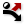Dispatch (Dispatch)
Sets  >  List  >  Dispatch
Dispatch the items in a list into two target lists.
Inputs
List (L)List to filter
Dispatch pattern (P)Dispatch pattern
Outputs
List A (A)Dispatch target for True values
List B (B)Dispatch target for False values
component.

We now have all the nodes but the surfaces, that are next to each other, share the same vertices. Thus, by dismantling the surfaces, we got vertices that have the same coordinates as vertices from an adjacent surface. Thus, we use Cull Duplicates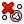Cull Duplicates (CullPt)
Vector  >  Point  >  Cull Duplicates
Cull points that are coincident within tolerance
Inputs
Points (P)Points to operate on
Tolerance (T)Proximity tolerance distance
Outputs
Points (P)Culled points
Indices (I)Index map of culled points
Valence (V)Number of input points represented by this output point
and flatten its input P to end up with a list of unique nodes that will serve for modeling the joints.

#### 3

##### Select the surrounding surfaces

Next, we select the surfaces that connect to the identified nodes. The vertices of each grid cell are separated in lists and this will help us to get the right surfaces. We connect a Sort ListSort List (Sort)
Sets  >  List  >  Sort List
Sort a list of numeric keys.
Inputs
Keys (K)List of sortable keys
Values A (A)Optional list of values to sort synchronously
Outputs
Keys (K)Sorted keys
Values A (A)Synchronous values in A
component to the Point In BrepPoint In Brep (BrepInc)
Surface  >  Analysis  >  Point In Brep
Test whether a point is inside a closed brep
Inputs
Brep (B)Brep for inclusion test
Point (P)Point for inclusion test
Strict (S)If true, then the inclusion is strict
Outputs
Inside (I)True if point is on the inside of the Brep.
detection from before and append a List Item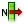List Item (Item)
Sets  >  List  >  List Item
Retrieve a specific item from a list.
Inputs
List (L)Base list
Index (i)Item index
Wrap (W)Wrap index to list bounds
Outputs
Item (i)Item at {i'}
on which we Reverse input L. Now, if one of the vertices is within the box, the value 1 for True is forwarded. Using DispatchDispatch (Dispatch)
Sets  >  List  >  Dispatch
Dispatch the items in a list into two target lists.
Inputs
List (L)List to filter
Dispatch pattern (P)Dispatch pattern
Outputs
List A (A)Dispatch target for True values
List B (B)Dispatch target for False values
with both inputs flattened will sort through the surfaces and select those which are connected to our nodes.

#### 4

##### Select the connected curves

To find the curves which are connected to the nodes, we grab the edges E from the dismantled grid cells and attach an End PointsEnd Points (End)
Curve  >  Analysis  >  End Points
Extract the end points of a curve.
Inputs
Curve (C)Curve to evaluate
Outputs
Start (S)Curve start point
End (E)Curve end point
component on which we graft both outputs. We now use Point In BrepPoint In Brep (BrepInc)
Surface  >  Analysis  >  Point In Brep
Test whether a point is inside a closed brep
Inputs
Brep (B)Brep for inclusion test
Point (P)Point for inclusion test
Strict (S)If true, then the inclusion is strict
Outputs
Inside (I)True if point is on the inside of the Brep.
to check whether one of the end points is within our box. By using Sort ListSort List (Sort)
Sets  >  List  >  Sort List
Sort a list of numeric keys.
Inputs
Keys (K)List of sortable keys
Values A (A)Optional list of values to sort synchronously
Outputs
Keys (K)Sorted keys
Values A (A)Synchronous values in A
and List ItemList Item (Item)
Sets  >  List  >  List Item
Retrieve a specific item from a list.
Inputs
List (L)Base list
Index (i)Item index
Wrap (W)Wrap index to list bounds
Outputs
Item (i)Item at {i'}
(with input L reversed) we again forward 1 for True to a DispatchDispatch (Dispatch)
Sets  >  List  >  Dispatch
Dispatch the items in a list into two target lists.
Inputs
List (L)List to filter
Dispatch pattern (P)Dispatch pattern
Outputs
List A (A)Dispatch target for True values
List B (B)Dispatch target for False values
component with both inputs flattened.

We now have all the edges that are completely or partially in the box, but because adjacent cells have equal edges, there are some duplicate ones. We can remove duplicate curves by getting their midpoint as a Point On Curve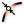Point On Curve (CurvePoint)
Curve  >  Analysis  >  Point On Curve
Evaluates a curve at a specific location
and then use Cull DuplicatesCull Duplicates (CullPt)
Vector  >  Point  >  Cull Duplicates
Cull points that are coincident within tolerance
Inputs
Points (P)Points to operate on
Tolerance (T)Proximity tolerance distance
Outputs
Points (P)Culled points
Indices (I)Index map of culled points
Valence (V)Number of input points represented by this output point
to get the indices of the duplicates. It’s important that we right-click Cull DuplicatesCull Duplicates (CullPt)
Vector  >  Point  >  Cull Duplicates
Cull points that are coincident within tolerance
Inputs
Points (P)Points to operate on
Tolerance (T)Proximity tolerance distance
Outputs
Points (P)Culled points
Indices (I)Index map of culled points
Valence (V)Number of input points represented by this output point
and select Leave One. A List ItemList Item (Item)
Sets  >  List  >  List Item
Retrieve a specific item from a list.
Inputs
List (L)Base list
Index (i)Item index
Wrap (W)Wrap index to list bounds
Outputs
Item (i)Item at {i'}
component will now do the sorting and we get the curves that will become the gridshell members.

#### 5

##### Find proper vectors for construction planes

All cells of the gridshell have a different orientation in space and the construction planes of the joints should deviate as little as possible from the adjacent surfaces. For this, we calculate the mean vector by adding the normal vectors of the corresponding cells.

Before we can add the vectors, we have to find the cells that are associated with a node. For this, we use Area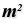Area (Area)
Surface  >  Analysis  >  Area
Solve area properties for breps, meshes and planar closed curves.
Inputs
Geometry (G)Brep, mesh or planar closed curve for area computation
Outputs
Area (A)Area of geometry
Centroid (C)Area centroid of geometry
to get the centers of the cells and then we use Closest Points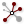Closest Points (CPs)
Vector  >  Point  >  Closest Points
Find closest points in a point collection.
Inputs
Point (P)Point to search from
Cloud (C)Cloud of points to search
Count (N)Number of closest points to find
Outputs
Closest Point (P)Point in [C] closest to [P]
CP Index (i)Index of closest point
Distance (D)Distance between [P] and [C](i)
to find the closest center points for each node. The number of the closest points N depends on the type of grid (triangular, hexagonal etc.) and on the position of the cell within the grid. We get this number from output V of the Cull DuplicatesCull Duplicates (CullPt)
Vector  >  Point  >  Cull Duplicates
Cull points that are coincident within tolerance
Inputs
Points (P)Points to operate on
Tolerance (T)Proximity tolerance distance
Outputs
Points (P)Culled points
Indices (I)Index map of culled points
Valence (V)Number of input points represented by this output point
component from step 2, because the number of neighboring cells equals the number of duplicate vertices.

We now have a separate list of associated surface indices for each node and a List ItemList Item (Item)
Sets  >  List  >  List Item
Retrieve a specific item from a list.
Inputs
List (L)Base list
Index (i)Item index
Wrap (W)Wrap index to list bounds
Outputs
Item (i)Item at {i'}
component will get us the actual surfaces. Then, we connect an Evaluate SurfaceEvaluate Surface (EvalSrf)
Surface  >  Analysis  >  Evaluate Surface
Evaluate local surface properties at a {uv} coordinate.
Inputs
Surface (S)Base surface
Point (uv){uv} coordinate to evaluate
Outputs
Point (P)Point at {uv}
Normal (N)Normal at {uv}
U direction (U)U direction at {uv}
V direction (V)V direction at {uv}
Frame (F)Frame at {uv}
and reparameterize input S. A PanelPanel
Params  >  Input  >  Panel
A panel for custom notes and text values
set to .5,.5 will evaluate the surface at its center. At output N we get the normal vectors and we add them with Mass Addition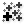Mass Addition (MA)
Maths  >  Operators  >  Mass Addition
Perform mass addition of a list of items
Inputs
Input (I)Input values for mass addition.
Outputs
Partial Results (Pr)List of partial results
. Also, we connect another Mass AdditionMass Addition (MA)
Maths  >  Operators  >  Mass Addition
Perform mass addition of a list of items
Inputs
Input (I)Input values for mass addition.
Outputs
Partial Results (Pr)List of partial results
to output U to get the mean orientation of each node.

#### 6

##### Create solids for joints

The next task is to create the construction planes with the just created vectors: First we create planes perpendicular to the mean normal vectors with Plane Normal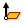Plane Normal (Pl)
Vector  >  Plane  >  Plane Normal
Create a plane perpendicular to a vector.
Inputs
Origin (O)Origin of plane
Z-Axis (Z)Z-Axis direction of plane
Outputs
Plane (P)Plane definition
. At input O we connect the nodes from step 2 as origins and flatten input Z to which we connect the normal vectors. Then, we append an Align PlaneAlign Plane (Align)
Vector  >  Plane  >  Align Plane
Perform minimal rotation to align a plane with a guide vector
Inputs
Plane (P)Plane to straighten
Direction (D)Straightening guide direction
Outputs
Plane (P)Straightened plane
Angle (A)Rotation angle
component and connect the mean orientation vector with input D flattened.

Now that we have our construction planes, we will create a ring that the grid members can later attach to. In a PanelPanel
Params  >  Input  >  Panel
A panel for custom notes and text values
with Multiline Data turned off, we write the inner and outer radius of our ring (here 0.10 and 0.12) and draw matching circles with CircleCircle (Cir)
Curve  >  Primitive  >  Circle
Create a circle defined by base plane and radius.
Inputs
Plane (P)Base plane of circle
Outputs
Circle (C)Resulting circle
(graft input R). Explode Tree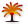Explode Tree (BANG!)
Sets  >  Tree  >  Explode Tree
Extract all the branches from a tree
Inputs
Data (D)Data to explode
Outputs
Branch 0 (-)All data inside the branch at index: 0
Branch 1 (-)All data inside the branch at index: 1
and Ruled Surface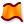Ruled Surface (RuleSrf)
Surface  >  Freeform  >  Ruled Surface
Create a surface between two curves.
Inputs
Curve A (A)First curve
Curve B (B)Second curve
Outputs
Surface (S)Ruled surface between A and B
will create a flat ring that will serve as base for the solids.

The axes of the grid members will meet the ring at half height, so we have to extrude it upwards and downwards. The direction of the extrusion vectors is given by the mean normal vectors and we have to adjust their length. A panel with 0.05 will give us a value for half of the height and Negative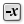Negative (Neg)
Maths  >  Operators  >  Negative
Compute the negative of a value.
Inputs
Value (x)Input value
Outputs
Result (y)Output value
will create the counterpart. Both values are connected to an Amplitude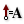Amplitude (Amp)
Vector  >  Vector  >  Amplitude
Set the amplitude (length) of a vector.
Inputs
Vector (V)Base vector
Amplitude (A)Amplitude (length) value
Outputs
Vector (V)Resulting vector
component on which we graft input A and flatten input V. Now, each vector will be converted into two short vectors. But, we need to use Flip Matrix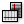Flip Matrix (Flip)
Sets  >  Tree  >  Flip Matrix
Flip a matrix-like data tree by swapping rows and columns.
Inputs
Data (D)Data matrix to flip
Outputs
Data (D)Flipped data matrix
to adjust the data sorting for the rings.

To put it all together, we use an Extrude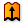Extrude (Extr)
Surface  >  Freeform  >  Extrude
Extrude curves and surfaces along a vector.
Inputs
Base (B)Profile curve or surface
Direction (D)Extrusion direction
Outputs
Extrusion (E)Extrusion result
component and it generates the solids from the rings. We have to graft and simplify input B and simplify input D to get matching data streams. Because we have two extrusions for each node, one upward and one downward, we can combine them to a single Brep with Solid Union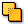Solid Union (SUnion)
Intersect  >  Shape  >  Solid Union
Perform a solid union on a set of Breps.
Inputs
Breps (B)Breps to union
Outputs
Result (R)Union result
.

#### 7

##### Shorten length of grid members

We will continue with the curves from step 4. The goal is to shorten them, so that they end on the center lines of the rings. In this example, we will cut off 0.11 from each end. After retrieving their initial Length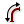Length (Len)
Curve  >  Analysis  >  Length
Measure the length of a curve.
Inputs
Curve (C)Curve to measure
Outputs
Length (L)Curve length
, we use Subtraction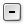Subtraction (A-B)
Maths  >  Operators  >  Subtraction
Mathematical subtraction
Inputs
A (A)First operand for subtraction
B (B)Second operand for subtraction
Outputs
Result (R)Result of subtraction
to calculate the end points on one side. On the other side, we need to start at 0.11. But we need this value for each line. Thus, we have to use Longest List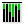Longest List (Long)
Sets  >  List  >  Longest List
Grow a collection of lists to the longest length amongst them
Inputs
List (A) (A)List (A) to operate on
List (B) (B)List (B) to operate on
Outputs
to duplicate it as many times as our list of initial curves is long. Both values (start and end) are combined with Merge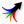Merge (Merge)
Sets  >  Tree  >  Merge
Merge a bunch of data streams
Inputs
Data 1 (D1)Data stream 1
Data 2 (D2)Data stream 2
Outputs
Result (R)Result of merge
on which graft the inputs. We now have lists that contain two values each.

To shatter the curves at these lengths, we have to calculate their curve parameters. For this, we use Evaluate Length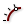Evaluate Length (Eval)
Curve  >  Analysis  >  Evaluate Length
Evaluate a curve at a certain factor along its length. Length factors can be supplied both in curve units and normalized units. Change the [N] parameter to toggle between the two modes.
Inputs
Curve (C)Curve to evaluate
Length (L)Length factor for curve evaluation
Normalized (N)If True, the Length factor is normalized (0.0 ~ 1.0)
Outputs
Point (P)Point at the specified length
Tangent (T)Tangent vector at the specified length
Parameter (t)Curve parameter at the specified length
and graft input C. We have to set input N to False, which states that we are working with the actual and not the normalized lengths. Next, we connect output t with a Shatter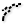Shatter (Shatter)
Curve  >  Division  >  Shatter
Shatter a curve into segments.
Inputs
Curve (C)Curve to trim
Parameters (t)Parameters to split at
Outputs
Segments (S)Shattered remains
component and also graft the curves that we connect to input C. The curves are now cut into three peaces. Because we only interested in the middle part, we use List ItemList Item (Item)
Sets  >  List  >  List Item
Retrieve a specific item from a list.
Inputs
List (L)Base list
Index (i)Item index
Wrap (W)Wrap index to list bounds
Outputs
Item (i)Item at {i'}
and set the index to 1. We now find the shortened curves at its output.

#### 8

##### Calculate vectors for construction planes

To create a section profile for each curve, we have to generate a construction plane. This should deviate as little as possible from the neighboring cells, so that the grid member has the same tilt to both adjacent surfaces (similar to step 5).

With Point On CurvePoint On Curve (CurvePoint)
Curve  >  Analysis  >  Point On Curve
Evaluates a curve at a specific location
we get the midpoint on each curve and then find the neighboring cells with Closest PointsClosest Points (CPs)
Vector  >  Point  >  Closest Points
Find closest points in a point collection.
Inputs
Point (P)Point to search from
Cloud (C)Cloud of points to search
Count (N)Number of closest points to find
Outputs
Closest Point (P)Point in [C] closest to [P]
CP Index (i)Index of closest point
Distance (D)Distance between [P] and [C](i)
. The number of points N to look for is given by output V of Cull DuplicatesCull Duplicates (CullPt)
Vector  >  Point  >  Cull Duplicates
Cull points that are coincident within tolerance
Inputs
Points (P)Points to operate on
Tolerance (T)Proximity tolerance distance
Outputs
Points (P)Culled points
Indices (I)Index map of culled points
Valence (V)Number of input points represented by this output point
from step 4. The same way as in step 5, we use Evaluate SurfaceEvaluate Surface (EvalSrf)
Surface  >  Analysis  >  Evaluate Surface
Evaluate local surface properties at a {uv} coordinate.
Inputs
Surface (S)Base surface
Point (uv){uv} coordinate to evaluate
Outputs
Point (P)Point at {uv}
Normal (N)Normal at {uv}
U direction (U)U direction at {uv}
V direction (V)V direction at {uv}
Frame (F)Frame at {uv}
, reparameterize its input S, set .5,.5 as uv-coordinate and calculate the mean vector with Mass AdditionMass Addition (MA)
Maths  >  Operators  >  Mass Addition
Perform mass addition of a list of items
Inputs
Input (I)Input values for mass addition.
Outputs
Partial Results (Pr)List of partial results
attached to output N.

#### 9

##### Create solids for grid members

Next, we will give body to the grid members. By using Perp Frame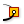Perp Frame (PFrame)
Curve  >  Analysis  >  Perp Frame
Solve the perpendicular (zero-twisting) frame at a specified curve parameter.
Inputs
Curve (C)Curve to evaluate
Parameter (t)Parameter on curve domain to evaluate
Outputs
Frame (F)Perpendicular curve frame at {t}
and reparameterizing its input C, we can create planes perpendicular to the curves. The location on the curves (input t) is set to their starting point with 0. These planes are not yet aligned with our surfaces and we need to use Align PlaneAlign Plane (Align)
Vector  >  Plane  >  Align Plane
Perform minimal rotation to align a plane with a guide vector
Inputs
Plane (P)Plane to straighten
Direction (D)Straightening guide direction
Outputs
Plane (P)Straightened plane
Angle (A)Rotation angle
together with our vectors from step 8. Input P has to be grafted so that the data structures match.

The actual section profile is drawn as a Rectangle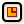Rectangle (Rectangle)
Curve  >  Primitive  >  Rectangle
Create a rectangle on a plane
Inputs
Plane (P)Rectangle base plane
X Size (X)Dimensions of rectangle in plane X direction.
Y Size (Y)Dimensions of rectangle in plane Y direction.
Outputs
Rectangle (R)Rectangle
Length (L)Length of rectangle curve
that has the X and Y dimensions -0.045 To 0.045. This way, our curves remains the axes of our members. We then use Sweep1Sweep1 (Swp1)
Surface  >  Freeform  >  Sweep1
Create a sweep surface with one rail curve.
Inputs
Rail (R)Rail curve
Sections (S)Section curves
Miter (M)Kink miter type (0=None, 1=Trim, 2=Rotate)
Outputs
Brep (S)Resulting Brep
to create solids along the full length of our curves. Again, we have to do same grafting for matching the data structures.

#### 10

##### Solve intersection for joints and grid members

In the steps before, we have created joints and grid members as solids, but they still interpenetrate. We can now either trim the joints with the grid members or the grid members with the joints by using the Solid Difference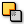Solid Difference (SDiff)
Intersect  >  Shape  >  Solid Difference
Perform a solid difference on two Brep sets.
Inputs
Breps A (A)First Brep set
Breps B (B)Second Brep set
Outputs
Result (R)Difference result
component. This depends on the engineering of the joints. Likewise, more construction parts could be added in a similar fashion.

## Get the results

### Version Info

• Rhino 6.31
• Grasshopper 1.0.0007

## But I only have a mesh…

Let’s have a look at what has to be changed, if we initially started with a mesh instead of a surface. To quickly create a mesh, we use Mesh Surface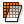Mesh Surface (Mesh UV)
Mesh  >  Util  >  Mesh Surface
Create a Surface UV mesh
Inputs
Surface (S)Surface geometry
U Count (U)Number of quads in U direction
V Count (V)Number of quads in V direction
Overhang (H)Allow faces to overhang trims
Equalize (Q)Equalize span length
Outputs
Mesh (M)UV Mesh
together with the grid cell dimensions from earlier. Face Boundaries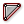Face Boundaries (FaceB)
Mesh  >  Analysis  >  Face Boundaries
Convert all mesh faces to polylines
Inputs
Mesh (M)Mesh for face boundary extraction
Outputs
Boundaries (B)Boundary polylines for each mesh face
will convert the mesh faces to polylines.

To rework step 1 (creating the capture box), we have to do a little more work to find a replacement for Evaluate SurfaceEvaluate Surface (EvalSrf)
Surface  >  Analysis  >  Evaluate Surface
Evaluate local surface properties at a {uv} coordinate.
Inputs
Surface (S)Base surface
Point (uv){uv} coordinate to evaluate
Outputs
Point (P)Point at {uv}
Normal (N)Normal at {uv}
U direction (U)U direction at {uv}
V direction (V)V direction at {uv}
Frame (F)Frame at {uv}
: We use Construct Point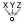Construct Point (Pt)
Vector  >  Point  >  Construct Point
Construct a point from {xyz} coordinates.
Inputs
X coordinate (X){x} coordinate
Y coordinate (Y){y} coordinate
Z coordinate (Z){z} coordinate
Outputs
Point (Pt)Point coordinate
together with sliders for the coordinates which will give us a point independent of our geometry. We can bring both together with Mesh Closest Point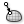Mesh Closest Point (MeshCP)
Mesh  >  Analysis  >  Mesh Closest Point
Finds the closest point on a mesh
Inputs
Point (P)Point to search from
Mesh (M)Mesh to search for closest point
Outputs
Point (P)Location on mesh closest to search point
Index (I)Face index of closest point
Parameter (P)Mesh parameter for closest point
and get the index of the mesh face closest to our point at output I. List ItemList Item (Item)
Sets  >  List  >  List Item
Retrieve a specific item from a list.
Inputs
List (L)Base list
Index (i)Item index
Wrap (W)Wrap index to list bounds
Outputs
Item (i)Item at {i'}
will find the matching curves from Face BoundariesFace Boundaries (FaceB)
Mesh  >  Analysis  >  Face Boundaries
Convert all mesh faces to polylines
Inputs
Mesh (M)Mesh for face boundary extraction
Outputs
Boundaries (B)Boundary polylines for each mesh face
.

To create a construction plane for our box, we use Is Planar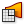Is Planar (Planar)
Surface  >  Analysis  >  Is Planar
Test whether a surface is planar
Inputs
Surface (S)Surface to test for planarity
Interior (I)Limit planarity test to the interior of trimmed surfaces
Outputs
Planar (F)Planarity flag of surface
Plane (P)Surface plane
which converts the polyline to a surface and evaluates its plane. We now have a base plane for our box from earlier.

What remains, is to find a substitution for Deconstruct BrepDeconstruct Brep (DeBrep)
Surface  >  Analysis  >  Deconstruct Brep
Deconstruct a brep into its constituent parts.
Inputs
Brep (B)Base Brep
Outputs
Faces (F)Faces of Brep
Edges (E)Edges of Brep
Vertices (V)Vertices of Brep
. This time, we work with the polylines instead of segmented surfaces. We can directly forward them to step 3, and they will be converted to surfaces with Surface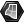Surface (Srf)
Params  >  Geometry  >  Surface
Contains a collection of generic surfaces
. Also, we break the Face Boundaries into lines with Explode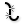Explode (Explode)
Curve  >  Util  >  Explode
Explode a curve into smaller segments.
Inputs
Curve (C)Curve to explode
Recursive (R)Recursive decomposition until all segments are atomic
Outputs
Segments (S)Exploded segments that make up the base curve
Vertices (V)Vertices of the exploded segments
and forward them (output S) to step 4. The ExplodeExplode (Explode)
Curve  >  Util  >  Explode
Explode a curve into smaller segments.
Inputs
Curve (C)Curve to explode
Recursive (R)Recursive decomposition until all segments are atomic
Outputs
Segments (S)Exploded segments that make up the base curve
Vertices (V)Vertices of the exploded segments
component will also give us the vertices of each face at output V. The start and end point of each face boundary are listed as two separate vertices, but they are in fact the same point. Thus, we use Cull Index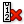Cull Index (Cull i)
Sets  >  Sequence  >  Cull Index
Cull (remove) indexed elements from a list.
Inputs
List (L)List to cull
Indices (I)Culling indices
Wrap (W)Wrap indices to list range
Outputs
List (L)Culled list
to remove the unnecessary point. The rest of step 2 will remain unchanged.

Now, every change is made to use the algorithm with meshes. It could be tweaked even more to use mesh faces for the calculation of the construction plane vectors. This optimization would be even more useful if additional Grasshopper Plugins would be used. For now, it’s fine for vanilla Grasshopper.

## Get the results

### Version Info

• Rhino 6.31
• Grasshopper 1.0.0007